﻿ A P-Series FormulaApplied Mathematics

p-ISSN: 2163-1409    e-ISSN: 2163-1425

2021;  11(2): 21-22

doi:10.5923/j.am.20211102.02

Received: Jun. 14, 2021; Accepted: Jul. 11, 2021; Published: Jul. 15, 2021### A P-Series Formula

Ameha Tefera Tessema

Commercial Bank of Ethiopia, Addis Ababa, Ethiopia

Correspondence to: Ameha Tefera Tessema, Commercial Bank of Ethiopia, Addis Ababa, Ethiopia.
 Email: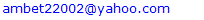Abstract

Let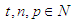such that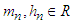then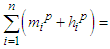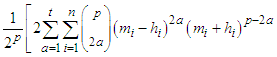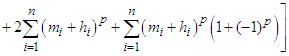Where p=2t+1 for all p odd Natural numbers(N), p=2t+2 for all p even natural numbers(N).

Keywords: Power series, Formula

Cite this paper: Ameha Tefera Tessema, A P-Series Formula, Applied Mathematics, Vol. 11 No. 2, 2021, pp. 21-22. doi: 10.5923/j.am.20211102.02.

1. Introduction

### 1. Introduction

In the early time fermat, a French number theorist, had stated that the equation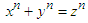has no solution in integers, if n>2 . The problem to find the exact value of z is enhanced as the number of terms increases rather than power two. Let us have n terms each of which has a common power p in the series then the series is called a p-series which is applied by the following theorem for p>2 which is also an answer for the claim of fermat.
Theorem 1. Let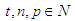such that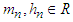then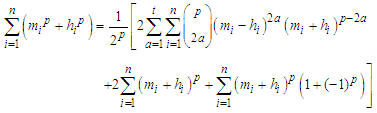Where p=2t+1 for all p odd Natural numbers(N), p=2t+2 for all p even natural numbers.
Proof. Let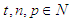such that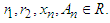if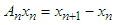wherethen multiplying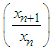up to n times we get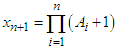, therefor we can write this expression to p power as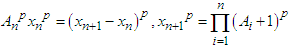If the binomial theorem for expansion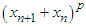.
Can be written as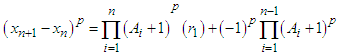Where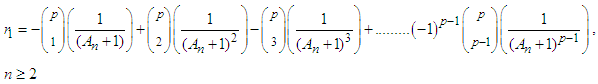,
Putting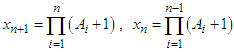and dividing both sides by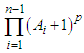we get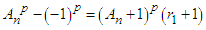(1)
The other way of writing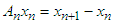is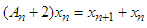which can be written to p power as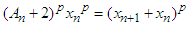If binomial theorem for expansion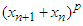can be written as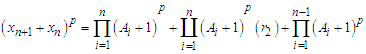Where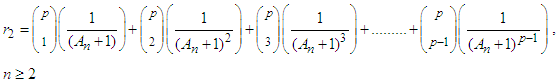,
Putting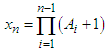in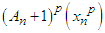and dividing both sides by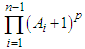we get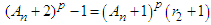(2)
Therefor, the summation of EQ(1) and EQ(2) can be put as follow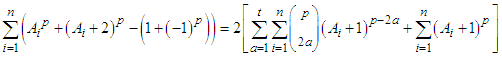Where p=2t+1 for all p odd natural numbers, whereas p=2t+2 for all p even natural numbers
Let put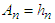and if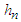be divided by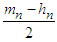such that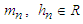(Real numbers) then we get the following result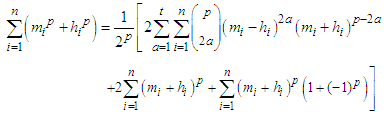This result led us to a conclusion that every couple terms which have common power can undergo this formula.

### References

  Gerhard Frey, The way to the proof of Fermat’s Last Theorem, Annales de la faculte des sciences de Toulouse, Vol. XVIII, 2009, pp.5-23.